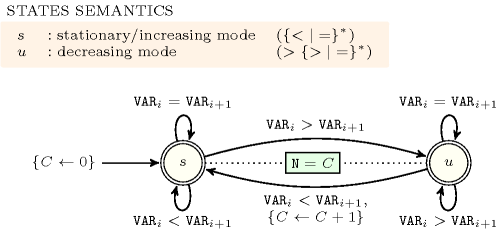### 3.7.24. Automaton with same input symbol

A constraint for which the catalogue provides an automaton belonging to the following category:

• Symbols of the alphabet are split in two categories: neutral ones and non-neutral ones.

• Non-neutral symbols correspond to symbols occurring on transitions between two distinct states, while neutral symbols correspond to all the other symbols of the alphabet.

• Self-loops labelled by a neutral symbol do not modify any counter.

• Ignoring transitions labelled by neutral symbols, every state has its incoming transitions labelled by the same non-neutral symbol.

• Ignoring transitions labelled by neutral symbols, outgoing transitions of a state are not labelled by the symbol associated with its incoming non-loop transitions.

For such automata we define the semantics of a state $s$ as the regular expression associated with the language fragment obtained from entering state $s$ to just before leaving state $s$.

##### Figure 3.7.6. Semantics of the states of the automaton of the $\mathrm{𝚟𝚊𝚕𝚕𝚎𝚢}$ constraintAs an example, consider the $\mathrm{𝚟𝚊𝚕𝚕𝚎𝚢}$ constraint and its automaton depicted by Figure 3.7.6. The alphabet $𝒜$ corresponds to the set of symbols $\left\{<,=,>\right\}$ from which $<$ and $>$ are non-neutral symbols (i.e., the symbols associated with the transitions between states $s$ and $u$), and $=$ is a neutral symbol. First there is no counter modification on all self-loops. If we remove the self-loops carrying the neutral symbol $=$ we have that:

• All incoming transitions in state $s$ are labelled by the non-symbol $<$, and all outgoing transitions from state $s$ are not labelled by $<$.

• All incoming transitions in state $u$ are labelled by the non-symbol $>$, and all outgoing transitions from state $u$ are not labelled by $>$.

The corresponding state semantics is given by the upper-leftmost box.